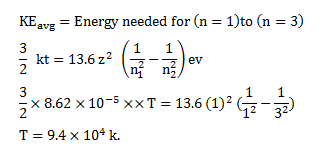# Find the temperature at which the average thermal kinetic energy is equal

Question:

Find the temperature at which the average thermal kinetic energy is equal to the energy needed to take a hydrogen atom from its ground state to $\mathrm{n}=3$ state. Hydrogen can now emit red light of wavelength $653.1 \mathrm{~nm}$. Because of Maxwellian distribution of speeds, a hydrogen sample emits red light at temperatures much lower than that obtained from this problem. Assume that hydrogen molecules dissociate into atoms.

Solution: